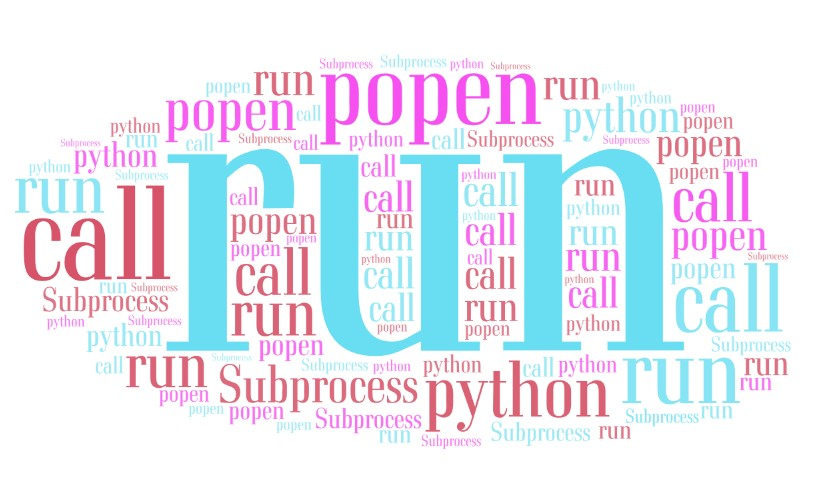# Python subprocess## What is subprocess?

A subprocess is a child process created by another process that executes a child program concurrently. Passing a string to a subprocess provides input for the subprocess to work with.

To use subprocess module write:

``````import subprocess
``````

This module allows you to spawn processes, connect to their input/output/error pipes, and obtain their return codes.

Type `dir(subprocess)` for more details.

Clearly `call()` and `run()` and `Popen()` are the often used methods.

`subprocess.call()` can run a subprocess and get just the return code.

`subprocess.run()` is more powerful than `call()`, it can return the return code, the output and the error.

`subprocess.Popen()` is even more powerful that `run()`, it can return whatever `run()` can and additional items such as process PID.

## Using `subprocess.call()`

This command waits for command to complete or to timeout, then returns the return code.

Example:

``````import subprocess
subprocess.call(["ls", "-all"])
# returns 0 (success)
``````

## Using `subprocess.run()`

Example:

``````import subprocess
from subprocess import PIPE
cp = subprocess.run(["ls","-all"], universal_newlines=True, stdout=PIPE, stderr=PIPE)
print(cp.args)
print(cp.returncode)
print(cp.stdout)
print(cp.stderr)
``````

Out:

``````['ls', '-all']
0
drwxr-xr-x 1 root root 4096 Nov 13 17:33 .
drwxr-xr-x 1 root root 4096 Nov 24 11:51 ..
drwxr-xr-x 1 root root 4096 Nov 13 17:33 tenor
drwxr-xr-x 1 root root 4096 Nov 13 17:33 baritone
drwxr-xr-x 1 root root 4096 Nov 13 17:33 bass
``````

Example: Error

``````import subprocess
from subprocess import PIPE
cp = subprocess.run(["ls", "soprano" ,"-all"], universal_newlines=True, stdout=PIPE, stderr=PIPE)

print(cp.args)
print(cp.returncode)
print(cp.stdout)
print(cp.stderr)
``````

Out:

``````['ls', 'soprano', '-all']
2

ls: cannot access 'soprano': No such file or directory
``````

## Using `subprocess.Popen()`

`subprocess.Popen()` has complex syntax vs. `call()` and `run()` but brings more control.

### Get the process id of the command

Example: PID

``````import subprocess
from subprocess import PIPE
p = Popen(["ls","-all"], stdout=PIPE, stderr=PIPE, universal_newlines=True)
pid = p.pid
pid # 313
``````

### Get the output and standard error

Example: Output or Error

``````import subprocess
from subprocess import PIPE
p = Popen(["ls","-all"], stdout=PIPE, stderr=PIPE, universal_newlines=True)
out, err = p.communicate()
print(out, err)
``````

### Write out to to a file based on file handle

``````import subprocess
from subprocess import Popen
from subprocess import PIPE
tmp = '/tmp/tmp.1.txt'
fh = open(tmp,'w+')
p = Popen(["ls","-all"], universal_newlines=True, stdout=fh, stderr=PIPE)
out, err = p.communicate()
print(out, err)
fh.close()
``````

Out:

``````None
``````

The output is inside the file:

``````\$> cat /tmp/tmp.1.txt

drwxr-xr-x 1 root root 4096 Nov 13 17:33 .
drwxr-xr-x 1 root root 4096 Nov 24 11:51 ..
drwxr-xr-x 1 root root 4096 Nov 13 17:33 tenor
drwxr-xr-x 1 root root 4096 Nov 13 17:33 baritone
drwxr-xr-x 1 root root 4096 Nov 13 17:33 bass
``````

### Waiting for the subprocess to finish

By default, calls to `Popen()` creates a subprocess in the background and don’t wait for it to terminate. You can wait for the subprocess to finish like this:

``````from subprocess import Popen
p = Popen(["ls","-all"])
p.wait()
``````

## Using `check_output()`

Example:

``````import subprocess
r = subprocess.check_output(["ls", "-all"], universal_newlines=True)
print(r)
``````

This function can also throw the exception in which case you may use `try` and `except` like this:

Example:

``````try:
result = subprocess.check_output(cmd, shell=True)
print(result)
except subprocess.CalledProcessError as error:
print(error)
``````

This method returns only the output, check the next method that works for both output and error.

## Using `getoutput()`

This method returns both the output in case no error and the error in case of error.

Example: Returns the output

``````import subprocess
r = subprocess.getoutput('ls -all')
print(r)
``````

Out:

``````drwxr-xr-x 1 root root 4096 Nov 13 17:33 .
drwxr-xr-x 1 root root 4096 Nov 24 11:51 ..
drwxr-xr-x 1 root root 4096 Nov 13 17:33 tenor
drwxr-xr-x 1 root root 4096 Nov 13 17:33 baritone
drwxr-xr-x 1 root root 4096 Nov 13 17:33 bass
``````

Example: Returns the error in case of error

``````import subprocess
r = subprocess.getoutput('ls soprano -all')
print(r)
``````

Out:

``````ls: cannot access 'soprano': No such file or directory
``````

## Run two commands in parallel

``````from subprocess import Popen
commands = ['command1', 'command2']
procs = [ Popen(i) for i in commands ]
for p in procs:
p.wait()
``````

## Run arbitrary many commands in parallel

``````%%time
from subprocess import Popen
from subprocess import PIPE
cmds_list = []
for s in range(100):
cmds_list.append(['sleep', str(2)])

procs_list = [Popen(cmd, stdout=PIPE, stderr=PIPE) for cmd in cmds_list]
for proc in procs_list:
proc.wait()
``````

Out:

``````CPU times: user 60.3 ms, sys: 303 ms, total: 363 ms
Wall time: 2.63 s
``````

In here we executed the command `sleep 2` for 100 times and that took 2.63 seconds. This means running the commands with `Popen()` is asynchronous.

## Another way of parallel execution

``````from concurrent.futures import ThreadPoolExecutor
import time

def exec_(cmd):
'''
single command run
command must be prepared as subprocess.call
'''
ret = subprocess.call(cmd)
if ret== 0:
print("success...")
else:
print("error")

def pull_run(parallel_jobs, cmds):
'''
run pull of jobs
input:
parallel_jobs: integer, how many jobs at once
cmds: list of commands
'''
futures = executor.map(exec_, cmds)
``````

We will run 10 times `sleep 2` but the max number of parallel jobs will be 4.

``````%%time
cmds = [['sleep', '2'] for i in range(10)]
pull_run(4, cmds)
``````

Out:

``````success...
success...
success...
success...
success...
success...
success...
success...
success...
success...
CPU times: user 19.4 ms, sys: 57.5 ms, total: 76.8 ms
Wall time: 6.07 s
``````

tags: subprocess & category: python